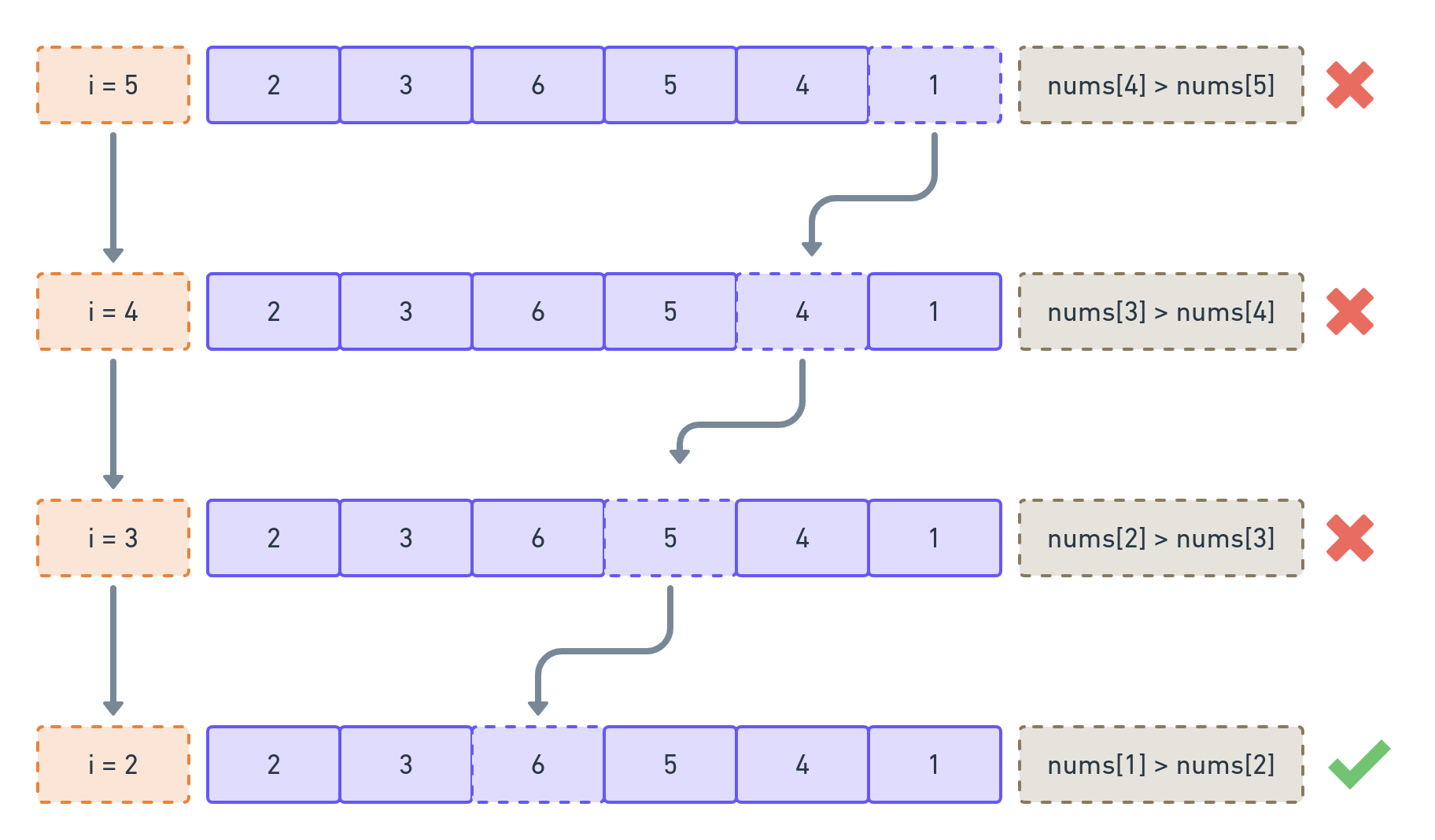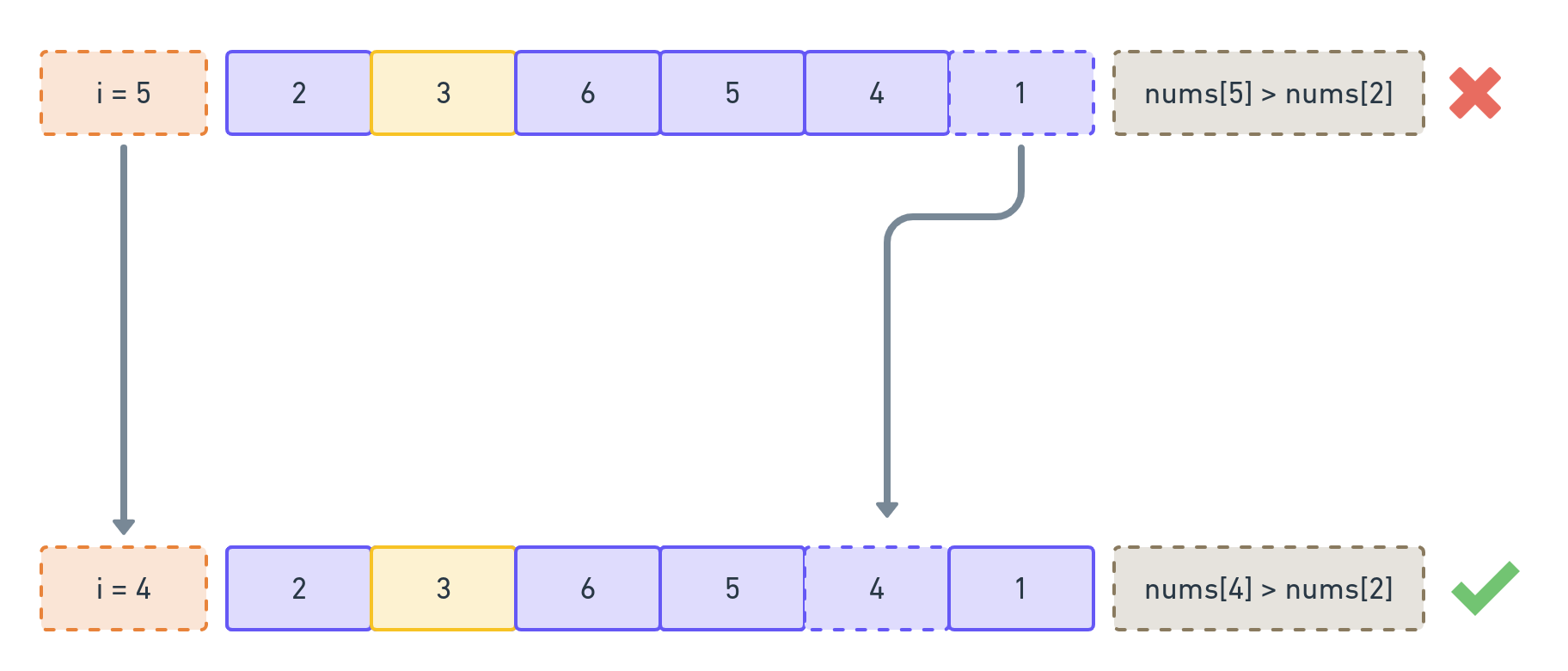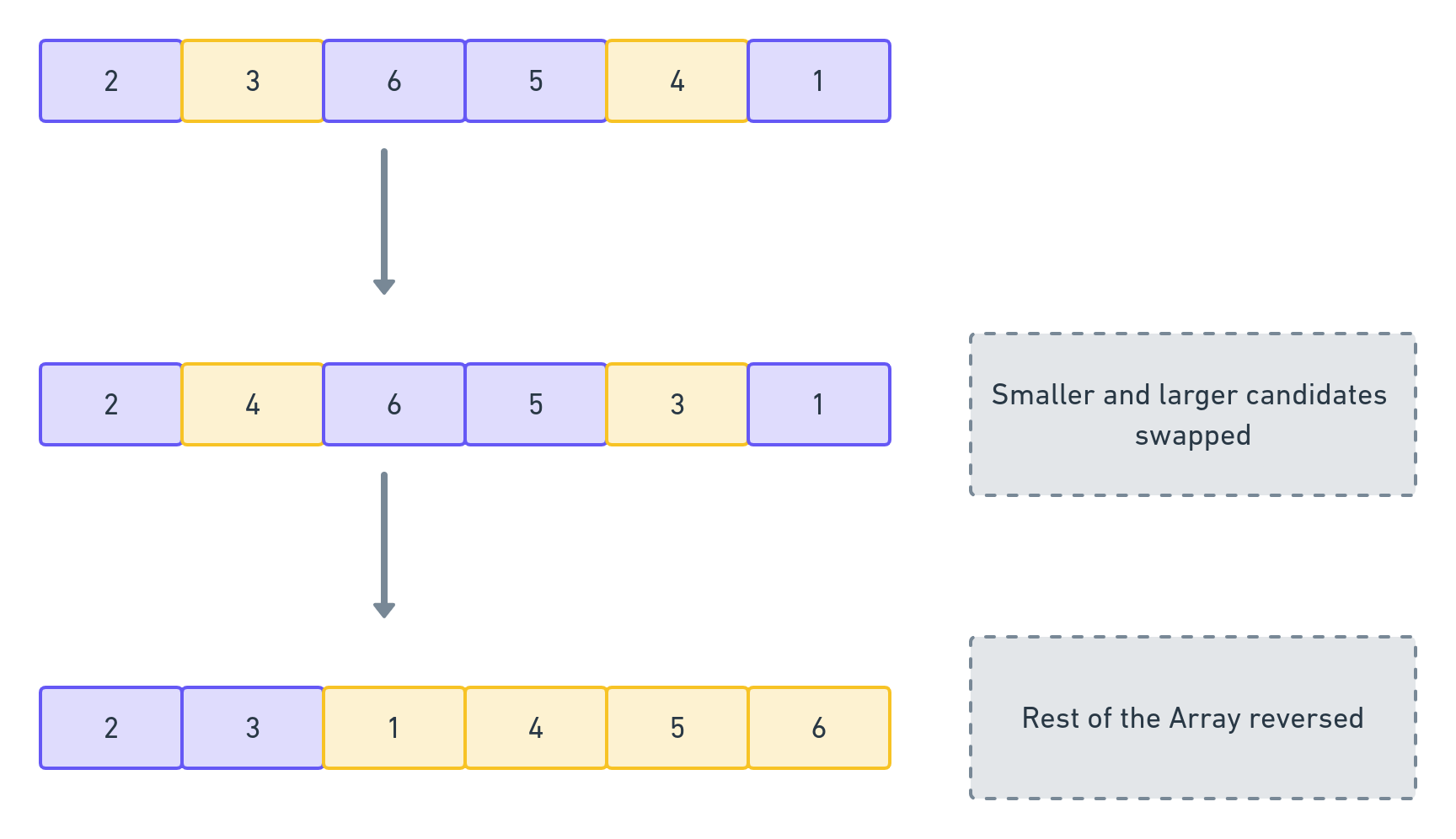# Finding the Lexicographically Next Permutation

October 27, 2021

In this post, I discuss the solution to the LeetCode Problem, titled Next Permutation.

## The Problem

Given an array of integers, `nums`, rearrange the elements of the array to produce the “lexicographically next greater permutation of numbers”.

If there is no such permutation possible (i.e., it is the greatest lexicographical permutation), the array should be rearranged to its “lowest possible order” , i.e., ascending order.

## What is lexicographical order?

Lexicographical order is a generalisation of the ‘aphpabetical order’ we see in dicitionaries. Words in a dictionary are ordered by the comparing alphabetical order of the letters, starting with the first (or left-most) place. By going left-to-right, we compare the corresponding letters of each index. We stop at the index, where the letters do not match. The word containing the letter which is alphabetically superior (at that particular index) gets a higher order.

For example, between the words, `peek` and `peak`, the word `peak` will be placed first, because `a` > `e` in the alphabetical order.

Not just dictionaries, lexicographical order is used in non-negative number systems as well. So, when we want to find the next permutation in Lexicographical order, it is similar to finding the next non-negative integer on the number scale (achieved solely by rearranging the existing digits). To simplify the explanation, I will use the example of integers, in the following part of this post.

## Solution Approach

In order to find the ‘next’ integer, we need to swap the positions of a specific pair of digits.

### Suitable Pair = Larger Candidate + Smaller Candidate

The pair of digits being replaced should not be equal to each other because otherwise we will end up with the original integer itself. Thus, one digit will be smaller (lets call it `Smaller Candidate`) and another will be greater (`Larger Candidate`).

Before proceeding further, we should note that the values carried by digits (in an integer) decrease from left-to-right. `2345` is actually `2 * 1000 + 3 * 100 + 4 * 10 + 5 * 1`. Thus, increasing `2` by `1` will create a much greater result, than increasing `4` with `1`.

Also note that, to find the ‘next’ integer, we need to find an integer, that:

• is greater than the given integer,
• is smaller than every other integer that is greater than the given integer.
• contains the exact same digits as the given integer (we can only replace the relative positions of the existing digits).

### Finding the Smaller Candidate

Now, lets assume, we somehow find the `Smaller Candidate`. The `Larger Candidate` can either be selected from its right-hand side or its left hand side. But because digits carry greater value as we go towards left, if we choose the `Larger Candidate` from the left of the `Smaller Candidate`, we will result with a smaller integer. Thus, the `Larger Candidate` needs to be selected from the digits on the left of the `Smaller Candidate`.

To achieve this, we need to know that there is at least one candidate to the right of the `Smaller Candidate` that is greater than `Smaller Candidate`.

Also, the `Smaller Candidate` should be the right-most digit, as long as we can guarantee that there is at least one digit to its right that is greater than itself. This is to ensure that we stick to the smallest-integer-greater-than rule.

Armed with this knowledge, we do a right-to-left sweep. At each index, we check if the digit to its left is smaller than itself, i.e., `nums[i - 1] < nums[i]`. The first pair that satisfies this, gives us the `Smaller Candidate`: namely, the `nums[i - 1]` candidate.

### Finding the Larger Candidate

Now, how do we choose the `Larger Candidate`? We know that the candidate immediately to the right of the `Smaller Candidate` is a potential candidate, because it is greater than the `Smaller Candidate`. But there may be another more suitable candidate on the right-hand side of the `Smaller Candidate`. So, we do another right-to-left sweep, and find out the first digit that is greater than the `Smaller Candidate`.

Why the first digit? Each digit to right of the `Smaller Candidate` will be arranged in descending order (if we go left-to-right from the `Smaller Candidate`). This is because, each of those digits failed the condition `nums[i - 1] < nums[i]`. In other words, while sweeping right-to-left, each succeeding element was either equal to or greater than the preceding digit. Thus, if we go right-to-left starting with the right-most digit, we get an ascending order, and the first digit that is larger than the `Smaller Candidate` will also be the smallest digit that is greater than the `Smaller Candidate`.

Now, that we know the `Smaller Candidate` and the `Larger Candidate`, we swap them.

### Re-arranging the Rest of the Array

We know that the digits next to the `Smaller Candidate` (now replaced by the `Larger Candidate`) are arranged in a descending order. This means that the right-most digit (which carries the maximum value) is also numerically the most superior. Because we need to find the smallest integer that is greater than the original integer, we re-arrange the digits to the right of the `Smaller Candidate` (now replaced by the `Larger Candidate`) in an ascending order.

### What happens when there is no Smaller Candidate?

If we are unable to find the `Smaller Candidate`, it would mean that we have completed the loop but could not find a single pair of digits that could satisfy: `nums[i - 1] < nums[i]`. In such a case, as required by the problem, we should re-arrange the entire array into ascending order. But, as every digit satisfied the aforesaid condition, they are all arranged in a descending order. So we should simply reverse their arrangement to arrive at the descending order.

## Example

Let’s take the example of `[2,3,6,5,4,1]`. First, we should find the `Smaller Candidate`, by comparing each pair of elements, starting with `1`.This condition is met when `i = 2` and the `Smaller Candidate` is `3` ( located at `[i - 1]`). Now, we do another right-to-left sweep. This time, we need to find the first element that is greater than `3`.This gives us `4`. We swap their positions and arrive at the array: `[2, 4, 6, 5, 3, 1]`. We need to now re-arrange the digits starting from the `2`nd index. This can be done by simply reversing their order.## Code

Here’s the code implementing the above approach:

``````var nextPermutation = function (nums) {
let i = nums.length - 1;
let smaller;
let reversed = false;
while (i > 0) {
if (nums[i - 1] < nums[i]) {
let replacingIndex = findSuccessor(i - 1);
let temp = nums[index - 1];
nums[i - 1] = nums[replacingIndex];
nums[replacingIndex] = temp;
reverse(i);
reversed = true;
break;
}
i--;
}
if (!reversed) {
reverse(0);
}
function reverse(lo) {
let hi = nums.length - 1;
while (lo < hi) {
let temp = nums[hi];
nums[hi] = nums[lo];
nums[lo] = temp;
lo++;
hi--;
}
}
function findSuccessor(lo) {
for (let i = nums.length - 1; i > lo; i--) {
if (nums[i] > nums[lo]) {
return i;
}
}
}
//the problem does not require nums to be returned
};
``````